Next: Lenz's Law Up: Magnetic Induction Previous: Magnetic Induction

The phenomenon of magnetic induction plays a crucial role in three very useful electrical devices: the electric generator, the electric motor, and the transformer. Without these devices, modern life would be impossible in its present form. Magnetic induction was discovered in 1830 by the English physicist Michael Faraday. The American physicist Joseph Henry independently made the same discovery at about the same time. Both physicists were intrigued by the fact that an electric current flowing around a circuit can generate a magnetic field. Surely, they reasoned, if an electric current can generate a magnetic field then a magnetic field must somehow be able to generate an electric current. However, it took many years of fruitless experimentation before they were able to find the essential ingredient which allows a magnetic field to generate an electric current. This ingredient is time variation.

Consider a planar loop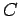of conducting wire of cross-sectional area. Let us place this loop in a magnetic field whose strengthis approximately uniform over the extent of the loop. Suppose that the direction of the magnetic field subtends an anglewith the normal direction to the loop. The magnetic flux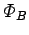through the loop is defined as the product of the area of the loop and the component of the magnetic field perpendicular to the loop. Thus,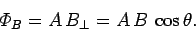(191)

If the loop is wrapped around itselftimes (i.e., if the loop hasturns) then the magnetic flux through the loop is simplytimes the magnetic flux through a single turn: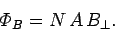(192)

Finally, if the magnetic field is not uniform over the loop, or the loop does not lie in one plane, then we must evaluate the magnetic flux as a surface integral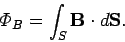(193)

Here,is some surface attached to. If the loop hasturns then the flux istimes the above value. The SI unit of magnetic flux is the weber (Wb). One tesla is equivalent to one weber per meter squared: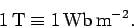(194)

Faraday discovered that if the magnetic field through a loop of wire varies in time then an emf is induced around the loop. Faraday was able to observe this effect because the emf gives rise to a current circulating in the loop. Faraday found that the magnitude of the emf is directly proportional to the time rate of change of the magnetic field. He also discovered that an emf is generated when a loop of wire moves from a region of low magnetic field-strength to one of high magnetic field-strength, and vice versa. The emf is directly proportional to the velocity with which the loop moves between the two regions. Finally, Faraday discovered that an emf is generated around a loop which rotates in a uniform magnetic field of constant strength. In this case, the emf is directly proportional to the rate at which the loop rotates. Faraday was eventually able to propose a single law which could account for all of his many and varied observations. This law, which is known as Faraday's law of magnetic induction, is as follows:

The emf induced in a circuit is proportional to the time rate of change of the magnetic flux linking that circuit.
SI units have been fixed so that the constant of proportionality in this law is unity. Thus, if the magnetic flux through a circuit changes by an amount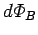in a time interval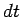then the emf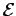generated in the circuit is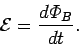(195)

There are many different ways in which the magnetic flux linking an electric circuit can change. Either the magnetic field-strength can change, or the direction of the magnetic field can change, or the position of the circuit can change, or the shape of the circuit can change, or the orientation of the circuit can change. Faraday's law states that all of these ways are completely equivalent as far as the generation of an emf around the circuit is concerned.Next: Lenz's Law Up: Magnetic Induction Previous: Magnetic Induction
Richard Fitzpatrick 2007-07-14